Latest Banking jobs   »

# Reasoning Ability Quiz For IDBI AM/Executive 2022- 20th June

Q1. If it is possible to make a meaningful word from 1st, 3rd, 5th, 7th and 11th letters of the word “EXASPERATION“, then which will be the 1st letter of that meaningful word? If more than one such meaningful words can be formed then ‘W’ is your answer. If no such meaningful word is formed, then ‘X’ is your answer.
(a) W
(b) A
(c) P
(d) O
(e) X

Reasoning Quiz For IDBI AM/Executive 2022 20th June CHECK IN HINDI

Q2. If it is possible to make a meaningful word from 3rd, 7th, 8th, 9th and 11th letters of the word “SURROUNDING“, then which will be the last letter of that meaningful word? If more than one such meaningful words can be formed then ‘W’ is your answer. If no such meaningful word is formed, then ‘X’ is your answer.
(a) W
(b) D
(c) I
(d) G
(e) X

Q3. If it is possible to make a meaningful word from 2nd, 5th, 6th, 8th, 9th, 11th and 12th letters of word “LOBORIOUSNESS“, then which will be the 6th letter of that meaningful word? If more than one such meaningful word can be formed then ‘Y’ is your answer. If no such meaningful word is formed, then ‘X’ is your answer.
(a) S
(b) U
(c) I
(d) Y
(e) X

Q4. If all the consonants of the word “SEPTEMBER” are replaced by their immediately preceding letter & vowels to their immediately following letter according to alphabetical series, then how many vowels will be there in the new rearranged word?
(a) Two
(b) Three
(c) Four
(d) Five
(e) None of the above

Q5. Which of the following letter will be the exactly middle letter, if all the letters of the word “VISHAKAPATNAM” are arranged in reverse alphabetical order from right to left?
(a) M
(b) K
(c) I
(d) H
(e) None of the above

Q6. Which of the following letter will be exactly middle letter, if the letters at odd positions are replaced by their succeeding letter in the word “PARALYSIS“?
(a) M
(b) A
(c) Y
(d) T
(e) None of the above

Q7. How many pairs of letters are there in the word “MELORA” which has as many letters between them as in the alphabetical series (both forward and backward direction)?
(a) One
(b) Two
(c) Three
(d) Four
(e) None of the above

Q8. How many pairs of letters are there in the word “SMYTTEN” which has as many letters between them as in the alphabetical series (both forward and backward direction)?
(a) None
(b) One
(c) Two
(d) Three
(e) Four

Q9. How many pairs of letters are there in the word “BLINKIT” which has as many letters between them as in the alphabetical series (both forward and backward direction)?
(a) None
(b) One
(c) Two
(d) Three
(e) Four

Q10. If each vowel of the word ‘FASHION’ is changed to its next letter and each consonant is changed to its previous letter according to English alphabetical series, after that, the alphabets thus formed are arranged in alphabetical order from left to right, then which of the following will be fifth letter from the left?
(a) P
(b) G
(c) M
(d) J
(e) None of the above

Q11. If odd placed digits are decreased by 1 & even placed digits are increased by 1 in the number “1357986420“, then find the difference between sum of odd and even placed digits after rearrangement?
(a) 7
(b) 8
(c) 9
(d) 10
(e) None of the above

Q12. If all the digits of the number “012345678” are increased by 1, then find sum of all even digits after rearrangement?
(a) 20
(b) 24
(c) 22
(d) 18
(e) None of the above

Q13. If all the digits of the number “987654456789” are decreased by 1, then find sum of all odd digits after rearrangement?
(a) 20
(b) 30
(c) 40
(d) 50
(e) None of the above

Q14. If all the digits of the number “014725836” are increased by 1, then find (sum of even placed digit value) / (sum of odd placed digit value) after rearrangement?
(a) 1/5
(b) 2/5
(c) 3/5
(d) 4/5
(e) None of the above

Q15. How many pairs of letters are there in the word “DIAGNOSTIC” which has as many letters between them as in the alphabetical series (both forward and backward direction)?
(a) None
(b) One
(c) Two
(d) Three
(e) Four

Solutions

S1. Ans. (d)
Sol. 1st, 3rd, 5th, 7th and 11th letters of the given word are E, A, P, R, O. So, the word OPERA can be formed. Hence, ‘O’ will be the first letter of the word.

S2. Ans. (b)
Sol. 3rd, 7th, 8th, 9th and 11th letters of the given word are R, N, D, I and G. So, the word GRIND can be formed. Hence, Last letter of the word is ‘D’.

S3. Ans. (b)
Sol. 2nd, 5th, 6th, 8th, 9th, 11th and 12th letters of the given word are O, R, I, U, S, E and S. So, the word formed is SERIOUS. Hence, the 6th letter of the word is ‘U’.

S4. Ans. (a)
Sol. Consonants are replaced by their previous letter and vowels to their next letter i.e.,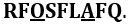There are two vowels in the new rearranged word. Hence, option (a) is the answer.

S5. Ans. (b)
Sol. If we arrange the letters in reverse alphabetical order from right to left, then we would get the following word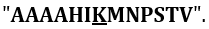So, the middle letter is K.

S6. Ans. (a)
Sol. If we replaced odd positioned letters with their succeeding letters, then we would get the respective word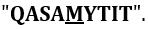So, the middle letter will be M.

S7. Ans. (a)
Sol.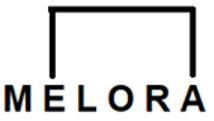S8. Ans. (a)
Sol. There is no pair.

S9. Ans. (c)
Sol.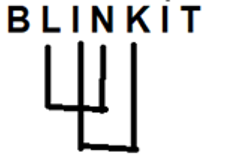S10. Ans. (c)
Sol. The rearranged word for FASHION is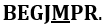Thus, M will be 5th from the left.

S11. Ans. (c)
Sol. The number is 1357986420. After rearrangement, the number become 0448895511.
Sum of all odd placed digits = 0 + 4 + 8 + 5 + 1 = 18
Sum of all even placed digits = 4 + 8 + 9 + 5 + 1 = 27
So, difference between odd & even placed digits = 27 – 18 = 9

S12. Ans. (a)
Sol. The number is 012345678. After rearrangement, the number becomes 123456789.
Sum of all even digits = 2 + 4 + 6 + 8 = 20

S13. Ans. (b)
Sol. The number is 987654456789. After rearrangement, the number becomes 876543345678.
Sum of all odd digits = 7 + 5 + 3 + 3 + 5 + 7 = 30

S14. Ans. (d)
Sol. The number is 014725836. After rearrangement, the number becomes 125836947.
Sum of odd placed digits = 1 + 5 + 3 + 9 + 7 = 25
Sum of even placed digits = 2 + 8 + 6 + 4 = 20
Required value = 20/25 = 4/5

S15. Ans. (e)
Sol.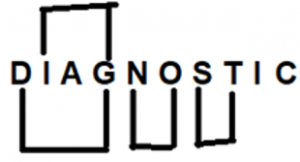#### Congratulations!